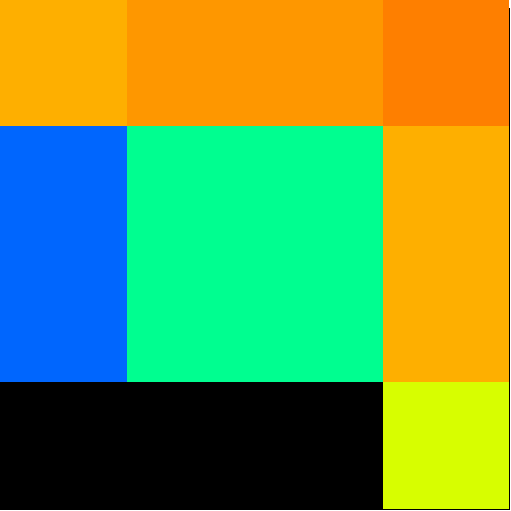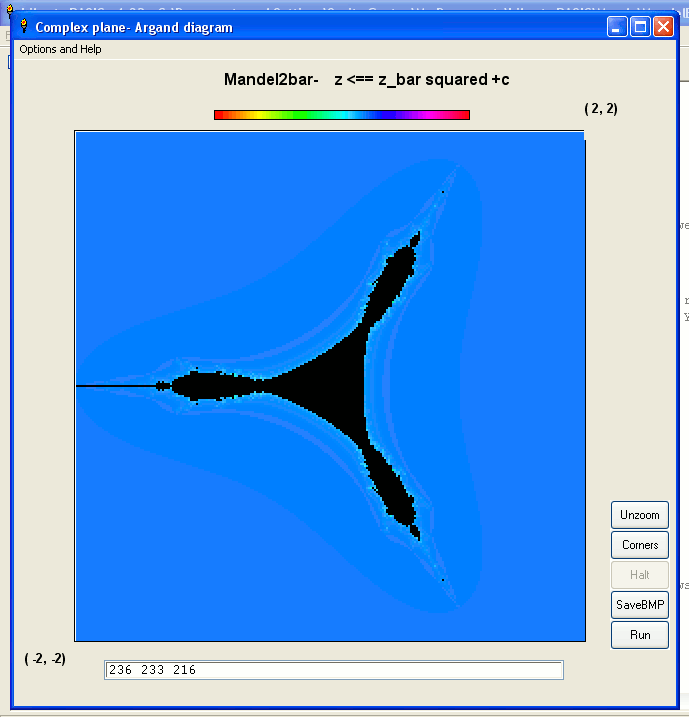# Mandelbrots and Mandelbars and other complex-plane setsThe infinite plane of all points referred to by two real-space dimensions ( x, y) holds many fun projects. Regarding the screen as two-dimensional, you can plot a representation of any function of x, or of x and y, and when colour-coded get lots of interesting patterns. Examples could be where y =f( x), or v =f( x, y). See link on my home page.
However another way of looking at the screen is as a view on a 'complex' plane, where the x axis is 'real' but the y-axis measures 'imaginary' numbers in a dimension with the interesting property that -1 DOES have a square root!
A lot of programmers must have coded the Mandelbrot set. It treats the x,y plane as holding complex numbers, represented as vectors from the origin to point ( x, iy), where z =x +iy. The plane is often called an Argand Diagram.
A vector z =( a, b) =a +ib, has length =sqrt( a^2 +b^2) and is at an angle theta =arctan( b /a).
When using the 'real' x,y plane, ( a +b)^2 =a^2 +2ab +b^2. Add vector a along the x axis and vector b along the y axis. You get a diagonal vector, an an in-between angle.
In the 'complex plane' however, ( a +ib)^2 is (a^2 -b^2) +2ab i. You are adding vector a (along the real axis) & vector b (along the 'imaginary' axis).
Since repeating up to 256 iterations at each point of a 512 by 512 lattice is slow, I code it so it first plots avery coarse & quick 4x4, and then keeps going back and redrawing at finer- and slower- resolutions. It can be left to run, and the end result left to save- or you can as at the top make an animation of perhaps an hours computing that replays at fast speed as an animated gif. ( Yet again, I use Imagemagick, or occasionally giftedmotion)
The other novelty is in regard to choosing colour coding of the set. I use pixel values read from colour gradient 256x10 bitmaps created in The Gimp. This gives 256 smoothly grading colours. Ideally, you want a bright, light colour immediately next to the set (large values of iterations-before-exit) and a different, pale colour near 1 ( ie a long way from the set). In between you can have just one sequence, but a repeated sequence also works well & creates an appearancve of higher resolution. The pixel colours are read into a lookup table of RGB values. I choose to use KCDan's method, which temporarily saves a 1x1 pixel bmp file and reads from it the pixel colour, before deleting it. Faster than you'd expect, because there isn't time for Windows to flush the buffer to 'real' hard drive file.Current version is Mandy.zip It has many known things to improve, but works and all files are in the zip.
The interface is still changing, and there are bugs & things to improve. Meanwhile, current 'look' is:-